# A Flurry of Facets

August 7, 2019
By

Want to share your content on R-bloggers? click here if you have a blog, or here if you don't.When I announced the last release of ggforce
I hinted that I would like to transition to a more piecemeal release habit and
avoid those monster releases that the last one was. True to my word, I am now
thrilled to announce that a new version of ggforce is available on CRAN for your
general consumption. It goes without saying that this release contains fewer
features and fixes than the last one, but those it packs are considerable so
let’s get to it.

## Build for gganimate

The gganimate package facilitates the creation of
animations from ggplot2 plots. It is build to be as general purpose as possible,
but it still makes a few assumptions about how the layers in the plot behaves.
Some of these assumptions where not met in a few of the ggforce geoms (the
technical explanation was that some stats and geoms stripped group information
from the data which trips up gganimate). This has been rectified in the new
version of ggforce and all geoms should now be ready for use with gganimate
(please report back if you run into any problems).

## Facets for the people

The remainder of the release centers around facets and a few geoms that has been

### Enter the matrix

The biggest news is undoubtedly the introduction of `facet_matrix()`, a facet
that allows you to create a grid of panels with different data columns in the
different rows and columns of the grid. Examples of such arrangements are known
as scatterplot matrices and pairs plots, but these are just a subset of the
general approach.

Before we go on I will, in the interest of full disclosure, mention that certain
types of scatterplot matrices have been possible for a long time. Most powerful
has perhaps been the `ggpairs()` function in GGally
that provides an API for pairs plots build on top of ggplot2. More low-level and
limited has been the possibility of converting the data to a long format by
stacking the columns of interest and using `facet_grid()`. The latter approach
requires that all columns of interest are of the same type and further moves a
crucial operation of the visualization out of the visualization API. The former
approach, while powerful, is a wrapper around ggplot2 rather than an extension
of the API. This means that you are limited to what the wrapper function
provides thus loosing the flexibility of the ggplot2 API. A plurality of choices
is good though, and I’m certain that there are rooms for all approaches to
thrive.

To show off `facet_matrix()` I’ll start with a standard use of scatterplot
matrices, namely plotting multiple components from a PCA analysis against each
other.

``````library(recipes)
# Data described here: https://bookdown.org/max/FES/chicago-intro.html

pca_on_stations <-
recipe(~ ., data = training %>% select(starts_with("l14_"))) %>%
step_center(all_predictors()) %>%
step_scale(all_predictors()) %>%
step_pca(all_predictors(), num_comp = 5) %>%
prep() %>%
juice()

pca_on_stations``````
``````## # A tibble: 5,698 x 5
##       PC1   PC2     PC3     PC4   PC5
##
##  1   1.37 4.41   0.347   0.150  0.631
##  2   1.86 4.50   0.618   0.161  0.523
##  3   2.03 4.50   0.569   0.0468 0.543
##  4   2.37 4.43   0.498  -0.209  0.559
##  5   2.37 4.13   0.422  -0.745  0.482
##  6 -15.7  1.23   0.0164 -0.180  1.04
##  7 -21.2  0.771 -0.653   1.35   1.23
##  8  -8.45 2.36   1.07   -0.143  0.404
##  9   3.04 4.30   0.555  -0.0476 0.548
## 10   2.98 4.45   0.409  -0.125  0.677
## # … with 5,688 more rows``````
``````library(ggforce)

ggplot(pca_on_stations, aes(x = .panel_x, y = .panel_y)) +
geom_point(alpha = 0.2, shape = 16, size = 0.5) +
facet_matrix(vars(everything()))``````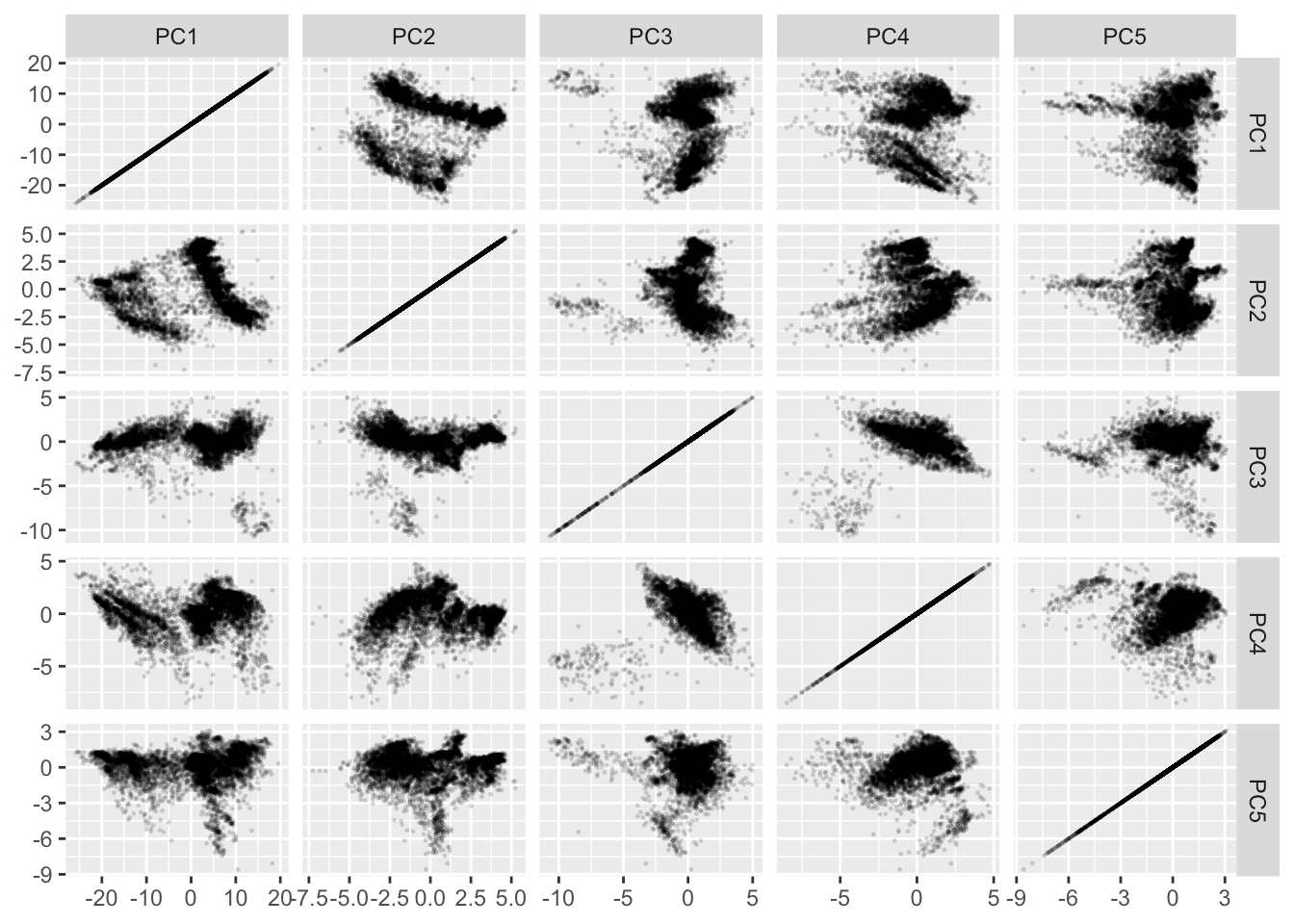Let’s walk through that last piece of code. We construct a standard ggplot using
`geom_point()` but we map x and y to `.panel_x` and `.panel_y`. These are
placeholders created by `facet_matrix()`. Lastly we add the `facet_matrix()`
specification. At a minimum we’ll need to specify which columns to use. For that
we can use standard tidyselect syntax as known from e.g. `dplyr::select()` (here
we use `everything()` to select all columns).

Now, the above plot has some obvious shortcomings. The diagonal is pretty
useless for starters, and it is often that these panels are used to plot the
distributions of the individual variables. Using e.g. `geom_density()` won’t
work as it always start at 0, thus messing with the y-scale of each row. ggforce
provides two new geoms tailored for the diagonal: `geom_autodensity()` and
`geom_autohistogram()` which automatically positions itself inside the panel
without affecting the y-scale. We’d still need to have this geom only in the
diagonal, but `facet_matrix()` provides exactly this sort of control:

``````ggplot(pca_on_stations, aes(x = .panel_x, y = .panel_y)) +
geom_point(alpha = 0.2, shape = 16, size = 0.5) +
geom_autodensity() +
facet_matrix(vars(everything()), layer.diag = 2)``````As the y-scale no longer affects the diagonal we’ll emphasize this by removing
the horizontal grid lines there:

``````ggplot(pca_on_stations, aes(x = .panel_x, y = .panel_y)) +
geom_point(alpha = 0.2, shape = 16, size = 0.5) +
geom_autodensity() +
facet_matrix(vars(everything()), layer.diag = 2, grid.y.diag = FALSE)``````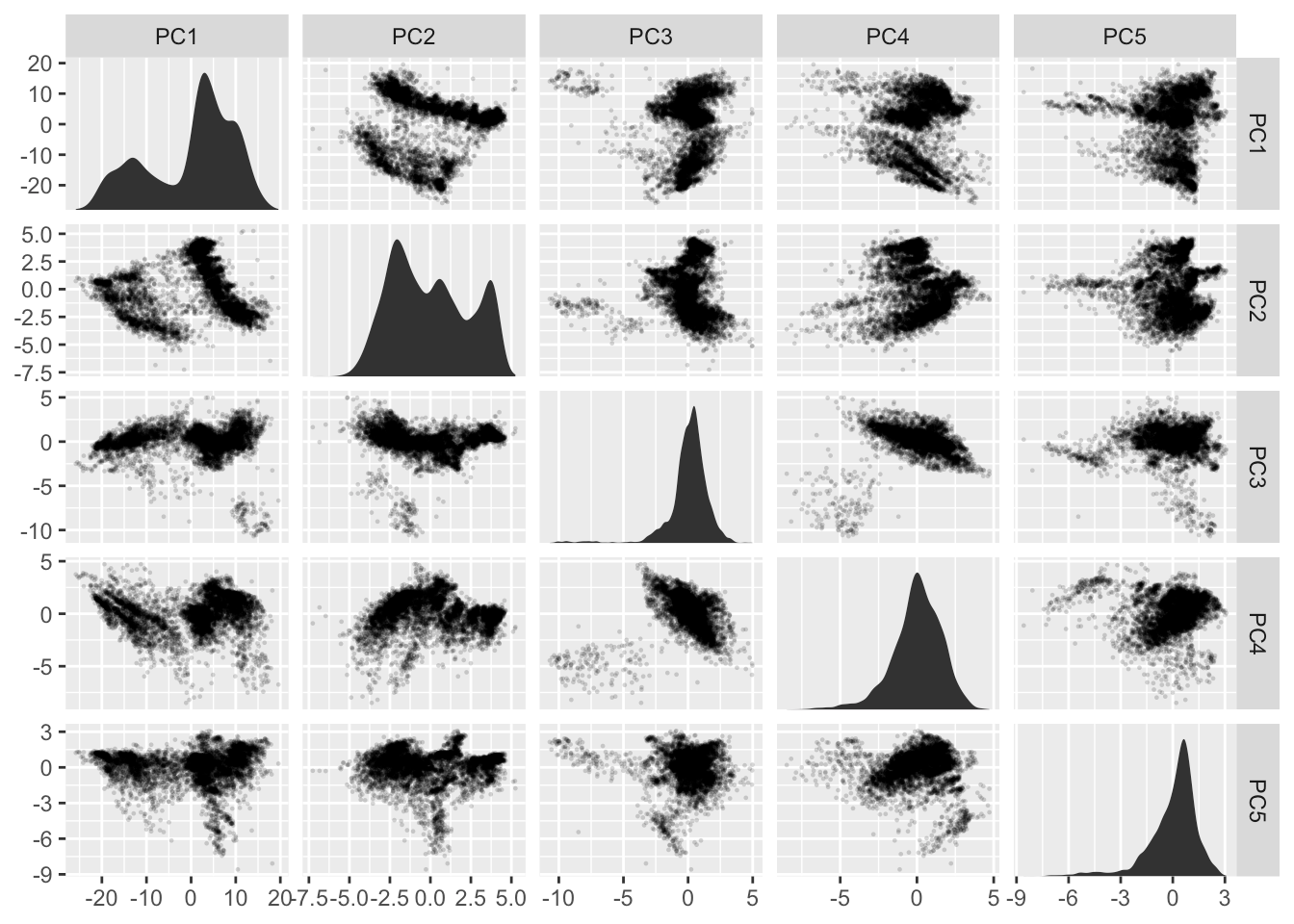There is still some redundancy left. As the grid is symmetrical the upper and
lower triangle shows basically the same (with flipped axes). We could add some
insight by using another geom in one of the areas that showed some summary

``````ggplot(pca_on_stations, aes(x = .panel_x, y = .panel_y)) +
geom_point(alpha = 0.2, shape = 16, size = 0.5) +
geom_autodensity() +
geom_density2d() +
facet_matrix(vars(everything()), layer.diag = 2, layer.upper = 3,
grid.y.diag = FALSE)``````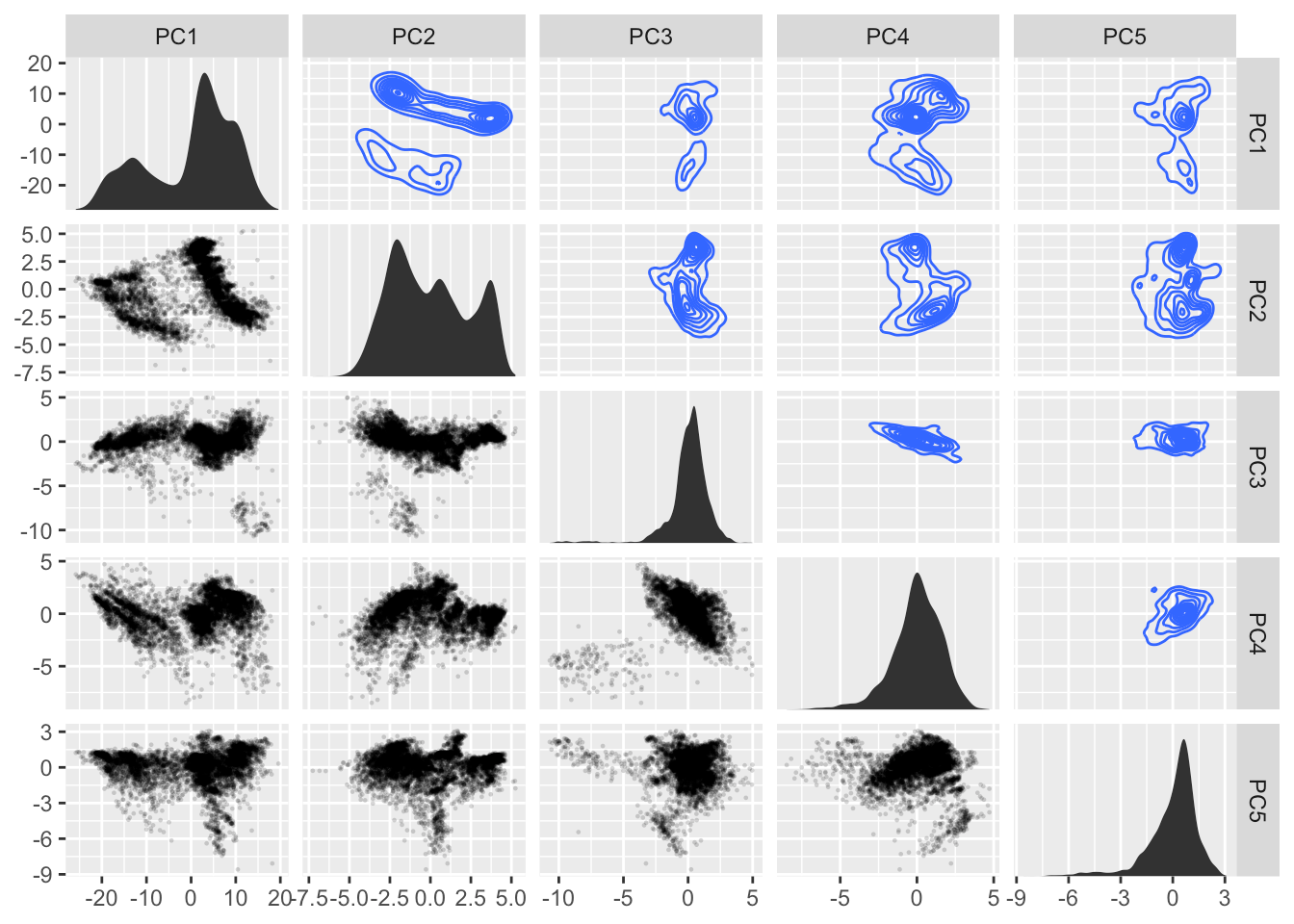While we could call this a day and be pretty pleased with ourselves, I’ll need
to show the final party trick of `facet_matrix()`. The above example was kind of
easy because all the variables were continuous. What if we had a mix?

``````ggplot(mpg, aes(x = .panel_x, y = .panel_y)) +
geom_point(shape = 16, size = 0.5) +
facet_matrix(vars(fl, displ, hwy))``````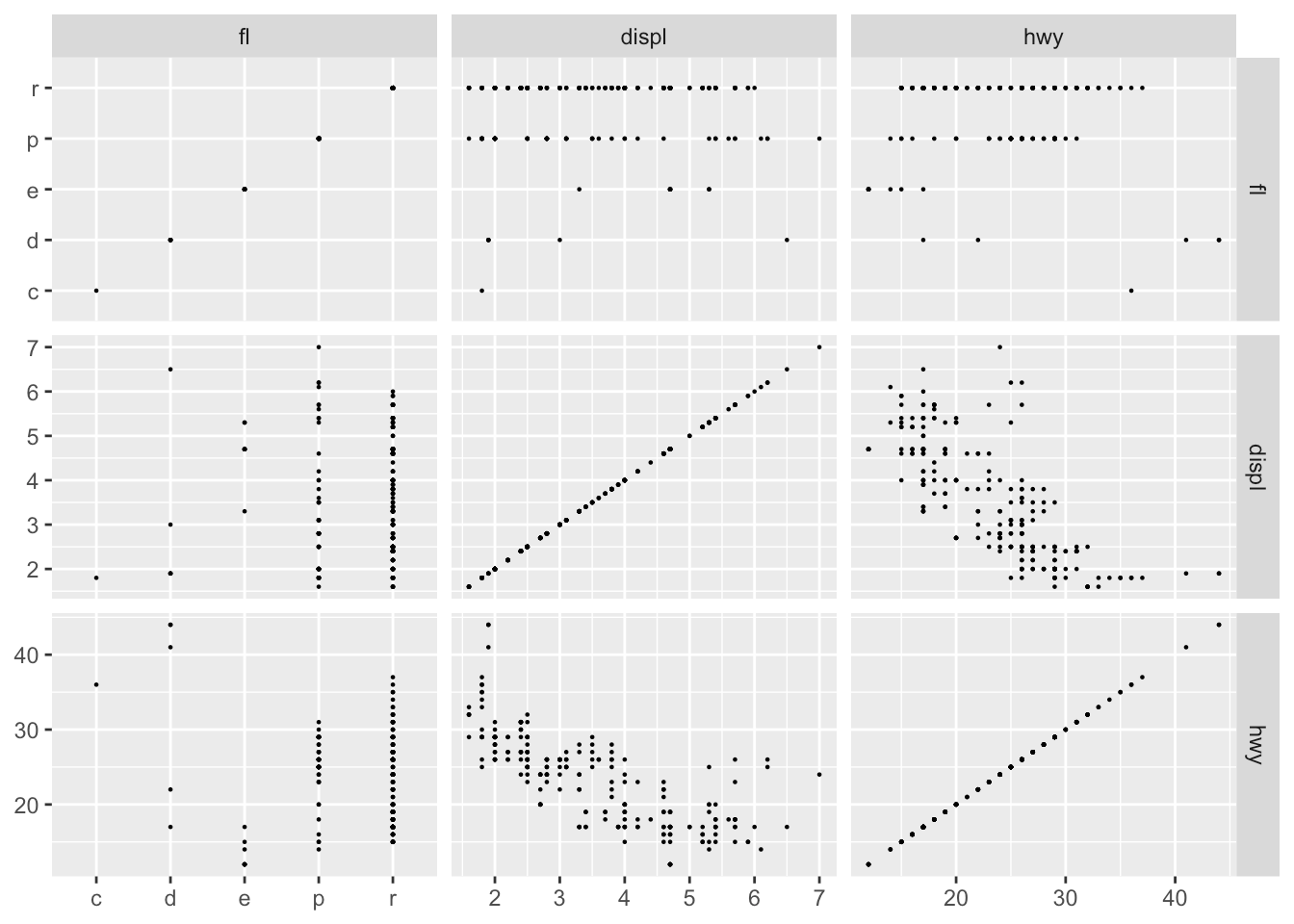As we can see `facet_matrix()` itself handles the mix of scale types quite well,
but `geom_point()` is not that telling when used on a mix of continuous and
discrete position scales. ggforce handles this by providing a new position
adjustment (`position_auto()`) that jitters the data based on the scale types.
For continuous vs discrete it does a sina-like jitter, whereas for discrete vs
discrete it jitters inside a disc (continuous vs continuous makes no jitter):

``````ggplot(mpg, aes(x = .panel_x, y = .panel_y)) +
geom_point(shape = 16, size = 0.5, position = 'auto') +
facet_matrix(vars(fl, displ, hwy))``````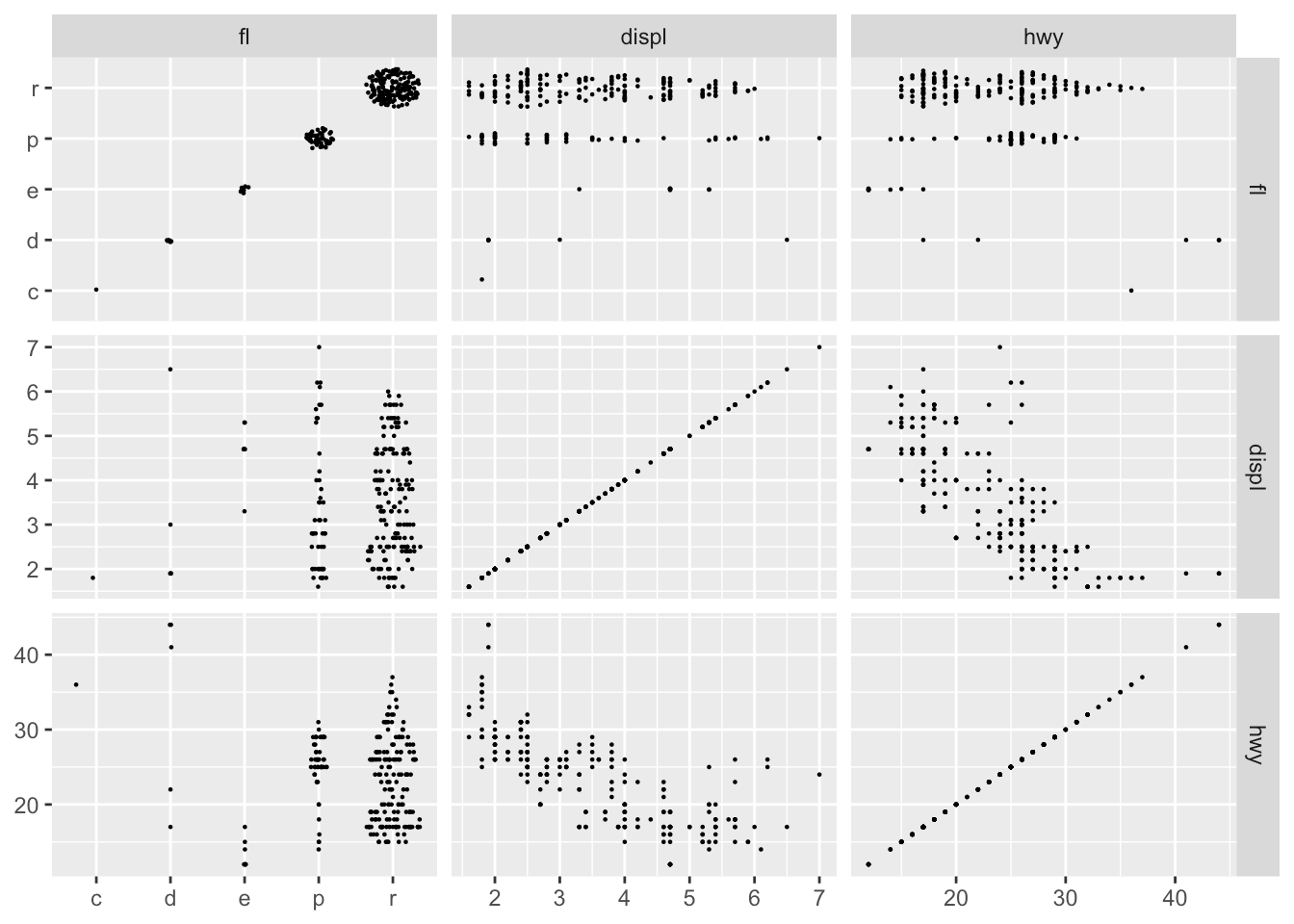`geom_autodensity()` and `geom_autohistogram()` also knows how to handle both
discrete and continuous data, so these can be used safely in all circumstances
(here also showing that you can of course also map other aesthetics):

``````ggplot(mpg, aes(x = .panel_x, y = .panel_y, fill = drv, colour = drv)) +
geom_point(shape = 16, size = 0.5, position = 'auto') +
geom_autodensity(alpha = 0.3, colour = NA, position = 'identity') +
facet_matrix(vars(fl, displ, hwy), layer.diag = 2)``````Lastly, if you need to use a geom that only makes sense with a specific
combination of scales, you can pick these layers directly, though you may end up
fiddling a bit to get all the right layers where you want them:

``````ggplot(mpg, aes(x = .panel_x, y = .panel_y, fill = drv, colour = drv)) +
geom_point(shape = 16, size = 0.5, position = 'auto') +
geom_autodensity(alpha = 0.3, colour = NA, position = 'identity') +
geom_smooth(aes(colour = NULL, fill = NULL)) +
facet_matrix(vars(fl, displ, hwy), layer.diag = 2, layer.continuous = TRUE,
layer.mixed = -3, layer.discrete = -3)``````The last example I’m going to show, is simply that you don’t have to create
symmetric grids. By default `facet_matrix()` sets the column selection to be the
same as the row selection, but you can overwrite that:

``````ggplot(mpg, aes(x = .panel_x, y = .panel_y)) +
geom_point(shape = 16, size = 0.5, position = 'auto') +
facet_matrix(vars(manufacturer, hwy), vars(drv, cty))``````As you can hopefully appreciate, `facet_matrix()` is maximally flexible, while
keeping the API of the standard use cases relatively clean. The lack of a
ggplot2-like API for plotting different variables against each others in a grid
has been a major annoyance for me, and I’m very pleased with how I finally
solved it—I hope you’ll put it to good use as well.

### Who needs two dimensions anyway?

The last new pack of facets are more benign, but something repeatedly requested.
`facet_row()` and it’s cousin `facet_col()` are one-dimensional mixes of
`facet_grid()` and `facet_wrap()`. They arrange the panels in a single row or
single column respectively (like setting `nrow` or `ncol` to `1` in
`facet_wrap()`), but by doing so allows the addition of a `space` argument as
known from `facet_grid()`. In contrast to using `facet_grid()` with a single
column or row, these new facets retain the `facet_wrap()` ability of having
completely separate scale ranges as well as positioning the facet strip

``````ggplot(mpg) +
geom_bar(aes(x = manufacturer)) +
facet_col(~drv, scales = 'free_y', space = 'free', labeller = label_both) +
coord_flip()``````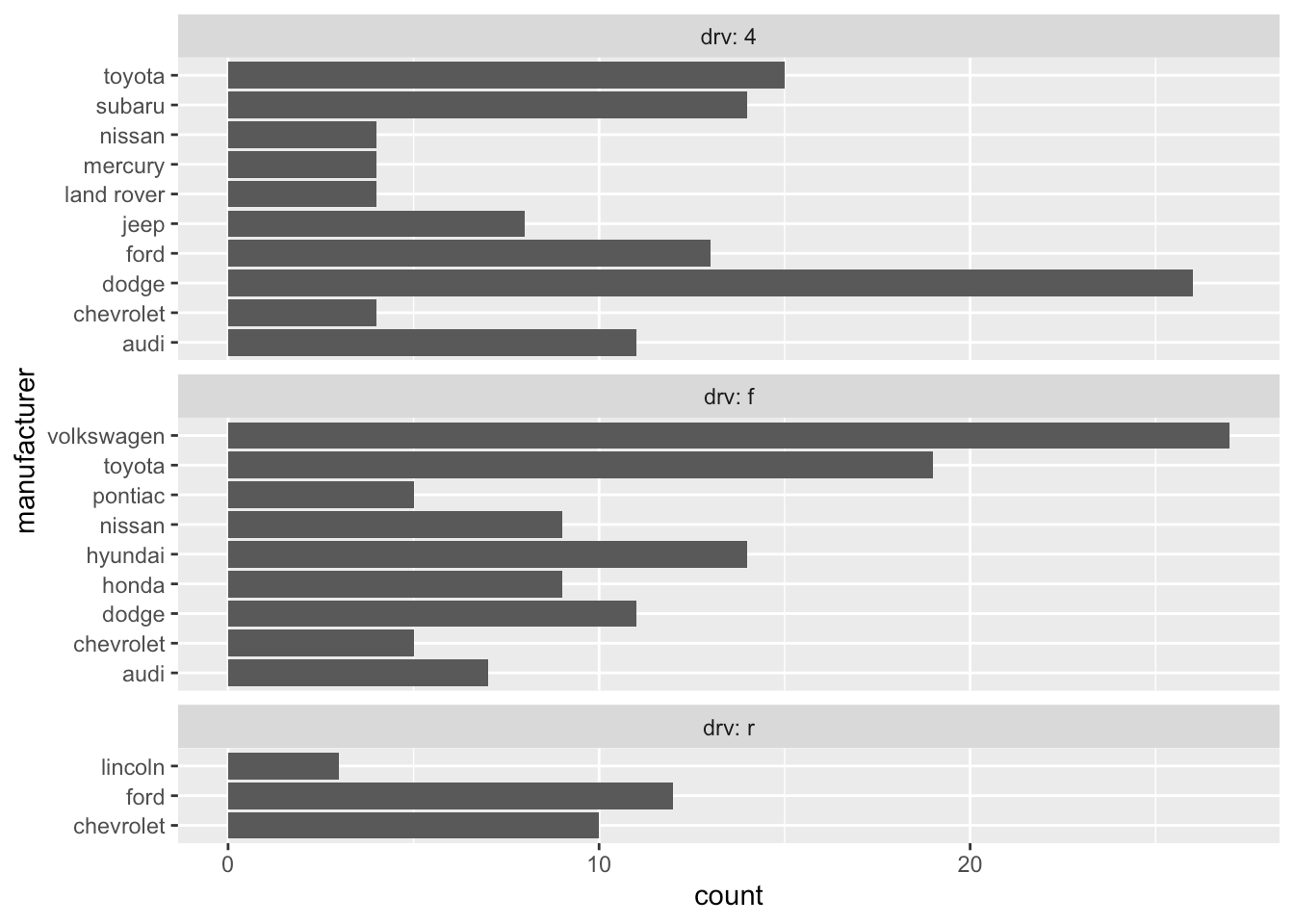So, these were the flurry of facets I was going to bring you today—I hope you’ll
put them to good use and create some awesome visualizations with them.

Next up: the next ggraph release!

R-bloggers.com offers daily e-mail updates about R news and tutorials about learning R and many other topics. Click here if you're looking to post or find an R/data-science job.
Want to share your content on R-bloggers? click here if you have a blog, or here if you don't.